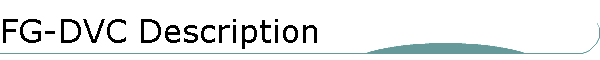# FG-DVC

THE FG-DVC MODEL DESCRIPTION AND VALIDATION

Advanced Fuel Research, Inc. (AFR) has developed strong expertise in the area of coal and biomass pyrolysis, in terms of both experimental research and pyrolysis modeling. An advanced coal-pyrolysis model, the FG-DVC model, has been under continuous development at AFR since the early 1980's. In what follows, the theoretical fundamentals of the FG-DVC model are discussed and selected examples of model validation are given. The discussion is provided for a coal feedstock, but other materials, such as biomass, solid wastes (e.g., scrap tires), can be treated in a similar fashion.

Statistical Network Models

The important processes in the early stages of pyrolysis are polymerization/depolymerization, cross-linking and gas formation, and these early processes determine the basic structure of the products [1, 2]. A statistical network model can be used to describe the pyrolysis of coal because of the polymeric / macromolecular nature of the feedstock. The geometrical structure of a polymer (whether it is chain-like or highly cross-linked) controls how it reacts under otherwise identical chemical reactions. Statistical models based on the geometrical structure are therefore required to predict the reactions of a polymer. AFR has developed such statistical models to describe the thermal decomposition of coal.

Many recent studies have proposed that coal can be thought of as having a macromolecular network structure to which concepts of cross-linked polymers can be applied [1-10]. These concepts have been employed to understand and model such properties of coal as: i) the insolubility; ii) the equilibrium swelling and penetration of solvents; iii) the viscoelastic properties; iv) similarities between the parent coal and products of hydrogenolysis, or mild oxidation; v) cross-linking during char formation [11-12]; and vi) the formation of coal tar in pyrolysis [13-15 ]. With the success of these concepts in describing coal properties, it is logical to extend macromolecular network concepts to completely describe the coal thermal decomposition behavior. This has been done at AFR by applying statistical methods to predict how the network behaves when subjected to thermally induced bond breaking, cross-linking, and mass transport processes [16-21].

The general model developed at AFR to describe coal thermal decomposition is called the FG-DVC model. It was described in several publications [19,20]. In developing the model, extensive experimental work was done with synthetic polymers to allow the study of bond breaking and mass transport in a chemically clean system [ 22-24]. The model combines two previously developed models, a functional group (FG) model [15,25,26] and a depolymerization-vaporization-cross-linking (DVC) model [16-18, 22-24]. The DVC subroutine is employed to determine the amount and molecular weight of macromolecular fragments, the lightest of which evolves as tar. The FG subroutine is used to describe the gas evolution and the elemental and functional group compositions of the tar and char. Cross-linking in the DVC subroutine is computed by assuming that this event is correlated with CO2 and CH4 evolutions predicted in the FG subroutine. The yield of rapidly released CO2 (which is related to coal rank and weathering) is the factor that controls the thermosetting or thermoplastic behavior of coals. For coals which exhibit thermoplastic behavior, the fluidity is limited by the cross-linking associated with the evolution of methyl groups.

The DVC Model - A simple example of the DVC model is shown in Figure. 1. In this model, the parent coal is represented as a two-dimensional network of monomers (condensed ring clusters) linked by strong and weak bridges. The monomers are represented by circles with molecular weights shown in each circle. The molecular weight distribution of the monomers is assumed to be Gaussian and is described by two parameters, Mavg (mean) and s (standard deviation). The monomers are linked to form unbranched oligomers of length l by breakable and non-breakable bridges (shown as horizontal single or double lines, respectively in Figure. 1a). mo crosslinks per gram are added (as vertical double lines in Figure. 1a) to connect the oligomers of length l so that the molecular weight between crosslinks, Mc corresponds to the value reported in the literature [ ] for coals of similar rank. The crosslinks form the branch points in the macromolecule. Unconnected "guest" molecules (the extract yield) are obtained by choosing the value of l .Figure 1.  Representation of a coal molecule in the DVC simulation and corresponding molecular weight distribution. In the molecule, the circles represent monomers (ring clusters and peripheral groups). The molecular weight shown by the numbers is the molecular weight of the monomer including the attached bridges. The single line bridges are breakable and can donate hydrogen.  The double line bridges are unbreakable and do not donate hydrogen.  The molecular weight distributions of the coal, tar, and chars are shown as histograms at the right.  The histogram is divided into tar and char with pyridine soluble and insoluble fractions.

The parameters Mc, l, Mavg, and s determine the molecular weight distribution of oligomers in the starting coal molecule. A histogram showing the distribution created by randomly picking monomers to form oligomers of length l, and randomly cross-linking them to achieve an average molecular weight between cross-links, Mc, is presented at the right of Figure. 1a. The distribution is divided into a pyridine-soluble portion below 3000 amu (light shading), and a pyridine-insoluble portion above 3000 amu (dark shading).

Figure 1b shows the molecule during pyrolysis. The rates for bridge breaking and cross-linking are determined from the FG model. Some bridges have broken, other bridges have been converted to unbreakable bridges by the abstraction of hydrogen to stabilize the free radicals, and new cross-links have been formed. To determine the change of state of the computer molecules during a time step, the number of cross-links formed is determined using the FG subroutine (see below), and passed to the DVC subroutine. These cross-links are distributed randomly throughout the char, assuming that the cross-linking probability is proportional to the molecular weight of the monomer. Then the DVC subroutine breaks the appropriate number of bridging bonds and calculates the quantity of tar evolved for this time step using the internal and external transport equations. The result is the coal molecule representation and the molecular weight distributions shown in Figure. 1b. The lighter "tar molecules," which leave the particle according to the transport equations, are cross hatched in Figure. 1b. A fraction of the donatable hydrogen is used to stabilize the free radicals formed by bridge breaking, creating two new methyl groups per bridge and the same fraction of breakable bridges is converted into (unbreakable) double bonds.

Figure 1c shows the final char, which is highly cross-linked with unbreakable bridges and has no remaining donatable hydrogen. The histogram now shows only tar and pyridine insoluble fractions. The extractables have been eliminated by tar formation and cross-linking. The output of the DVC subroutine is the molecular weight distribution in the coal, its time dependent transformation during devolatilization, and the evolution of tar determined by the transport of the lighter components.

The FG Model - The Functional Group (FG) model has been described in a number of publications [15,26,28]. It permits the detailed prediction of the composition of volatile species (gas yield, tar yield, and tar functional group and elemental composition) and of char (elemental and functional group composition). The original version employed coal-independent rates for the decomposition of individual functional groups in the coal and char to produce gas species. The approach was successful in predicting the evolution profiles and amounts of the volatile products [20,29]. More recently, it was found that rank-dependent kinetic rates for bridge breaking and cross-linking were required to make accurate predictions of coal fluidity, as discussed below and in Refs.  and .

Model Validation The model predictions have been compared to experiments on the pyrolysis of a Pittsburgh Seam coal [11,26,32,33], a North Dakota (Beulah, Zap) lignite [18,26], and the Argonne Premium coal samples [29,30]. Comparisons of the predictions with experiments are presented below.

Product Distribution - The ability of the FG model to predict the product distribution is illustrated in Figure. 2. The model predictions and experiments are described by Serio et al. . There is excellent agreement between the theory and data.

Extract Yields - Figure. 3 compares the FG-DVC predictions to the data of Fong et al.  on total volatile yield and extract yield as a function of temperature in pyrolysis at 0.85 atm . The experiments were performed in a heated-grid apparatus at heating rates of approximately 500 °C/sec, with variable holding times and rapid cool down. The predictions at the two higher temperatures (Figures. 3c and 3d) are in excellent agreement with the data. There is a discrepancy between the prediction and the data at early times for the two lower temperature cases (Figures. 3a and 3b). It is possible that the coal particles heat more slowly than the nominal temperatures given by Fong et al.

Cross-link Density - To examine the effect of coal rank on cross-linking, the volumetric swelling ratios (VSR) for North Dakota (Beulah, Zap) lignite, and Pittsburgh Seam bituminous coal were measured as a function of temperature at a heating rate of 0.5°C/sec. The VSR can be related to the cross-link density [11,27]. As discussed previously [18,20], under the assumption that the cross-linking reactions may also release gas species, the VSR was correlated with the observed evolution of gas species during pyrolysis. Correlations presented in references  and  show that, on a molar basis, the evolution of CO2 from the lignite and CH4 from the bituminous coal appear to have similar effects on the VSR. Reactions which form these gases leave behind free radicals which can be stabilized by cross-linking. The coals which undergo early cross-linking are less fluid, produce less tar, and produce lower molecular weight tar compared with coals which do not experience early cross-linking [16,17]. Assuming that one cross-link is formed for each CO2 or CH4 evolved from the char, the FG-DVC model predictions are in good agreement with the data, as discussed in references  and .Figure 2.  Pyrolysis results for North Dakota Lignite, 200 x 300 mesh, in the heated-tube reactor (HTR) at an equilibrium tube temperature of 800 ºC.  Solid lines in parts a-e are predictions of the Functional Group Model.  Solid lines in part f are predictions of the Time-Temperature Position Model.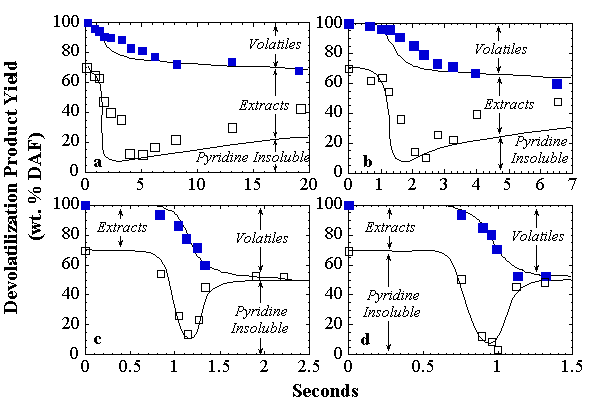Figure 3.  Comparison of FG-DVC model predictions (lines) with the data of Fong et al., 1986 (symbols) for Pittsburgh Seam coal: (a) 813 K @ 470 K/s; (b) 858 K @ 446 K/s; (c) 992 K @ 514 K/s; and (d) 1018 K @ 640 K/s.  P = 0.85 atm.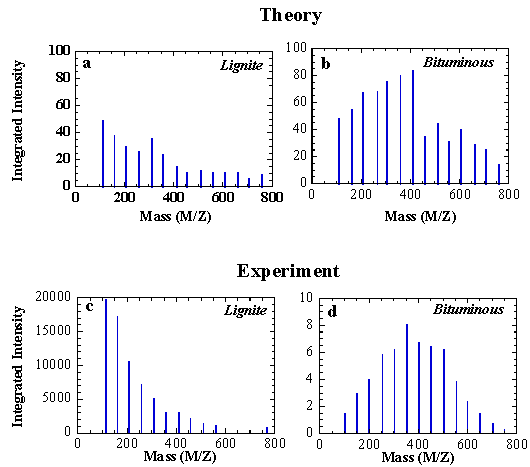Figure 4.  Comparison of predicted (parts a-b) and measured (parts c-d) tar molecular weight distributions for lignite and bituminous coals.  The experiments are performed by pyrolysis of coal samples in a FIMS apparatus.  Intensities have been summed over 50 amu intervals.

Molecular-Weight Distribution - A sensitive test of the model is the ability to predict the tar molecular weight distribution and its variations with rank, pressure, and heating rate. Figures 4c and 4d show experimental results for the Pittsburgh Seam bituminous coal and the Beulah Zap lignite pyrolyzed in the field ionization mass spectrometer (FIMS) apparatus . The data have been summed over 50 amu intervals. While the Pittsburgh bituminous coal shows a peak intensity at about 400 amu, the lignite peak is at 100 amu. The predicted average tar molecular weight distributions are in good agreement with FIMS data, as shown in Figure. 4a and 4b. Since both tar distributions are from the same monomer distribution, the enhanced drop-off in amplitude with increased molecular weight for the lignite, as compared with the bituminous coal, must be due to early cross-linking and transport effects in the lignite.

Pressure Effects - The FG-DVC model can also predict the effect of pressure on the tar molecular weight distribution and product yields, as described in reference . Pressure enters the model through the transport equations. The internal transport rate which is assumed to dominate, is inversely proportional to the ambient pressure Po. The reduced transport rate reduces the evolution rate of the heavier molecules. Therefore, the average molecular weight and the vaporization "cut-off" decrease with increasing pressure. The model was able to successfully predict tar and char yield variations from pyrolysis experiments done over a range of pressures from vacuum to ~100 atm .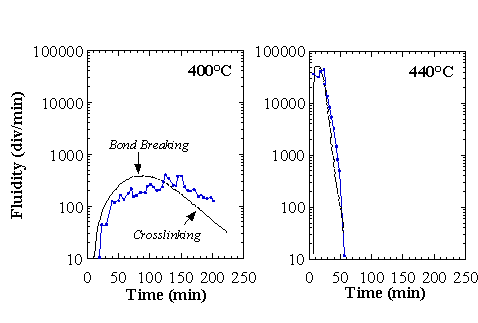Figure 5.  Comparison of the theory of fluidity for a high rank coal at constant temperatures of (a) 400 ºC, and (b) 440 ºC.  The experiment is for a coal with a 30% volatile matter content, and theory is for Upper Freeport coal.

Fluidity - A model for fluidity of coal has been developed based on a macromolecular network concept which is part of the FG-DVC model . The network model predicts the fraction of liquids and the average molecular weight of the liquids under the combined effects of bridge breaking and cross-linking. The empirical model for an inhomogeneous melt assumes the fluidity depends on the liquid fraction in the melt, on the viscosity of the fluid, and on the temperature. Good agreement has been obtained with data for four coals which cover five orders of magnitude in fluidity and several hundred degrees in temperature. This agreement is obtained with fixed parameters in the empirical fluidity equations but with adjustments of the bridge breaking and cross-linking rates which vary with the rank of the coal. The first example of the application of the model to predict fluidity is shown in Figure. 5. The data are from Oxley and Pitt , obtained by heating during an 11 minute period to constant temperatures of 400, 420, and 440 °C. The agreement is excellent because the model predicts the increase and decrease in fluidity due to bridge breaking and cross-linking, and fits data throughout two orders of magnitude change in fluidity, and over a 40 °C change in temperature.

Yields of Volatile Products and their Dependence on Coal-Rank, Heating Rate, and Pressure - A parity plot of predicted versus measured atmospheric pressure tar yields is shown in Figure. 6, which has been adapted from Zhao et al. . A perfect agreement between model predictions and experimental data corresponds to the diagonal line in the figure. It can be seen that all of the data indeed fall on or near the diagonal. It should be emphasized that the experimental data shown in Figure. 6 come from a number of independent investigations in which different experimental set-ups and conditions were used. In particular, the pyrolysis heating rate varied over several orders of magnitude (1-1000 K/s). Other examples of the good agreement between model predictions and experimental data are shown in Figures. 7-9.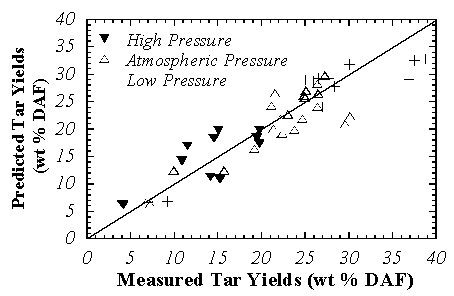Figure 6.  Comparison of the measured and the predicted tar yields for a number of coals.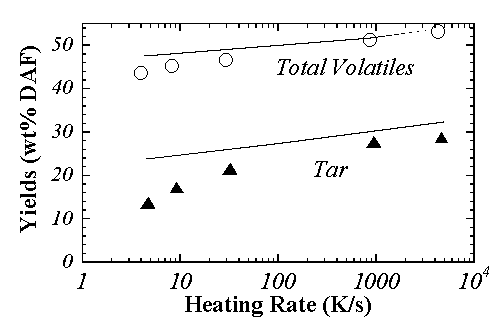Figure 7.  Variations of tar and total volatile yields as a function of heating rate at atmospheric pressure for an Illinois #6 coal.  The lines are predictions and the symbols are the data.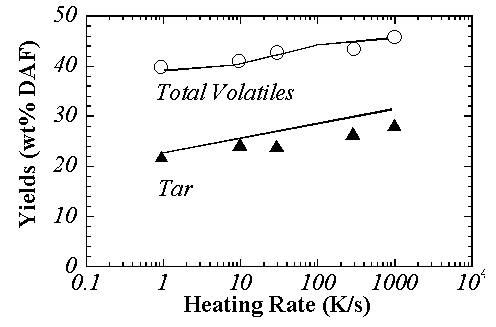Figure 8.  Variations of tar and total volatile yields as a function of heating rate at atmospheric pressure for a Linby coal.  The lines are predictions and the symbols are the data.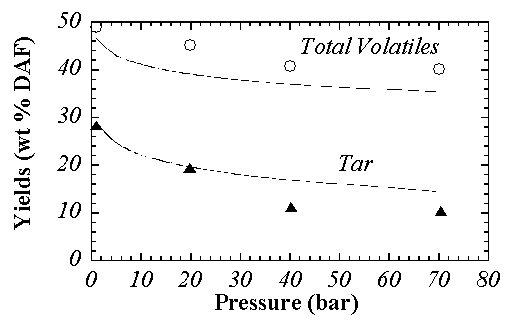Figure 9.  Variations of tar and total volatile yields as a function of pressure at 1000 K/s for an Illinois #6 coal.  The lines are predictions and the symbols are the data.

REFERENCES

1. Van Krevelen, D.W., Coal, Elsevier, Amsterdam, (1961).

2. Green, T.K., Kovac, J., and Larsen, J.W., Fuel, 63, 935 (1984).

3.  Green, T.K., Kovac, J., Larsen, J.W., Coal Structure, R.A. Meyers, Ed., Academic Press, (1982).

4. Lucht, L.M. and Peppas, N.A., Fuel, 66, 803, (1987).

5.  Lucht, L.M., Larsen, J.M., and Peppas, N.A., Energy & Fuels, 1, 56, (1987).

6.  Larsen, J.W., ACS Fuel Chem. Div. Preprints 30, (4), 444, (1985).

7. Green, T., Kovac, J., Brenner, D., and Larsen, J., Coal Structure, (R.A. Meyers, Ed.), Academic, NY, p 199, (1982).

8. Hall, P.J., Marsh, H., and Thomas, K.M., Fuel, 67, 863, (1988).

9. Sanada, Y. and Honda, H., Fuel, 45, 295, (1966).

10. Suuberg, E.M., Yoshi, O., and Deevi. S., ACS Div. Fuel Chem. Prepr. 33(1), 387, (1988).

11. Suuberg, E.M., Lee, D., and Larsen, J.W., Fuel, 64, 1668, (1985).

12. Suuberg, E.M., Unger, P.E., and Larsen, J.W., Energy & Fuels, 1, 305, (1987).

13. Solomon, P.R., New Approaches in Coal Chemistry, ACS Symp. Series 169, ACS, Washington, DC, 1981, pp. 61-71.

14. Solomon, P.R. and King, H.H., Fuel, 63, 1302, (1984).

15.  Solomon, P.R. and Hamblen, D.G., in Chemistry of Coal Conversion, (R.H.  Schlosberg, Editor), Plenum Press, NY, Chapter 5, p. 121, (1985).

16. Solomon, P.R., Squire, K.R., Carangelo, R.M., Proc, Int. Conf. Coal Science, Sydney, Australia, p. 945, 1985.

17. Solomon, P.R. and Squire, K.R., ACS Div. Fuel Chem. Prepr. 30, (4), 347, (1985).

18. Solomon, P.R., Hamblen, D.G., Deshpande, G.V. and Serio, M.A., Coal Science and Technology 11, (J.A. Moulijn, K.A. Nater, and H.A.G. Chermin, Eds.), Elsevier, Amsterdam, p. 601, (1987).

19. Solomon, P.R., Hamblen, D.G., Carangelo, R.M., Serio, M.A., and Deshpande, G.V., Combustion and Flame, 1988, 71, 137.

20. Solomon, P.R., Hamblen, D.G., Carangelo, R.M., Serio, M.A., and Deshpande, G.V., Energy & Fuels, 2, 405, (1988).

21. Solomon, P.R., Hamblen, D.G., Yu, Z.Z., and Serio, M.A., "Network Models of Coal Thermal Decomposition," Fuel 69, 754, (1990).

22. Solomon, P.R., Squire, K.R., Carangelo, R.M., ACS. Div. Fuel Chem. Prepr. 29(1), 10, (1984).

23. Squire, K.R., Solomon, P.R., Carangelo, R.M., and DiTaranto, M.B., Fuel 65, 833, (1986).

24. Squire, K.R., Solomon, P.R., DiTaranto, M.B., and Carangelo, R.M., ACS Div. Fuel Chem. Prepr. 30, (1), 386, (1985).

25. Solomon, P.R., Serio, M.A., Carangelo, R.M., and Markham, J.R., Fuel, 65, 182, (1986).

26. Serio, M.A., Hamblen, D.G., Markham, J.R., Solomon, P.R., Energy & Fuels, 1(2), 138, (1987).

27. Nelson, J.R., Fuel 62, 112, (1983).

28. Solomon, P.R., Hamblen, D.G., and Yu, Z.Z., "Network Models of Coal Thermal Decomposition", ACS Div. Fuel Chem. Prepr., 34(4), 1280, (1989).

29. Serio, M.A., Solomon, P.R., Yu, Z.Z., Deshpande, G.V., Hamblen, D.G., ACS Div. Fuel Chem. Prepr. 33(3), 91, (1988).

30. Serio, M.A., Solomon, P.R., Yu, Z.Z., and Deshpande, G.V., "An Improved Model of Coal Devolatilization", Presented at the Int. Conference on Coal Science, Japan (10/1989).

31.  Solomon, P.R., Best, P.E., Yu, Z.Z., and Deshpande, G.V., "A Macromolecular Network Model for Coal Fluidity", ACS Div. Fuel Chem. Prepr, 34(3), 895, (1989).

32.  Suuberg, E.M., Peters, W.A., and Howard, J.B., 17th Symp. (Int) on Combustion, The Combustion Institute, Pittsburgh, PA,1979, p. 117.

33. Fong, W.S., Peters, W.A., and Howard, J.B., Fuel 65, 251, (1986).

34. Carangelo, R.M., Solomon, P.R. and Gerson, D.J., "Application of TG-FTIR to Study Hydrocarbon Structure and Kinetics," Fuel 66, 960 (1987).

35.  St. John, G.A., Butrill, Jr., S.E. and Anbar, M., ACS Symposium Series 71, p. 223, (1978).

36.  Oxley, G.R. and Pitt, G.J., Fuel, 37, 19, (1958).

37.  Zhao, Y., Serio, M.A., Bassilakis, R. and Solomon, P.R., "A Method of Predicting Coal Devolatilization Behavior Based on the Elemental Composition," 25th Symposium (Int.) on Combustion, The Combustion Institute, Pittsburgh, PA, 1994, pp. 553-560.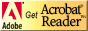[ Home ] [ Up ] [ Contact ] [ Company ] [ News & Events ] [ Careers ] [ Expertise ]